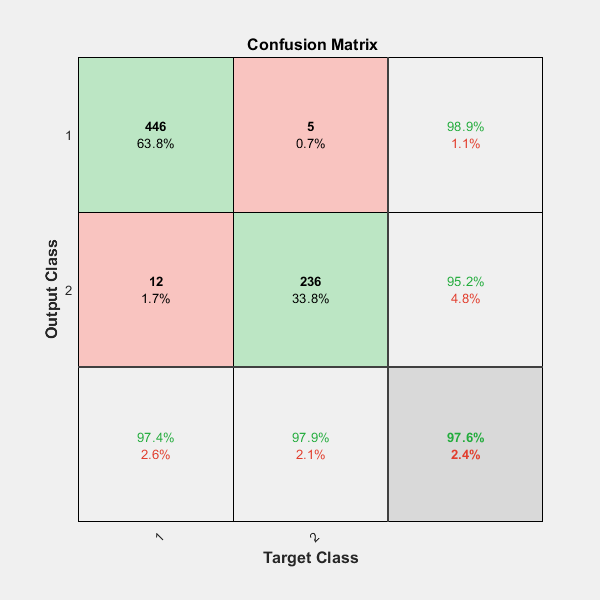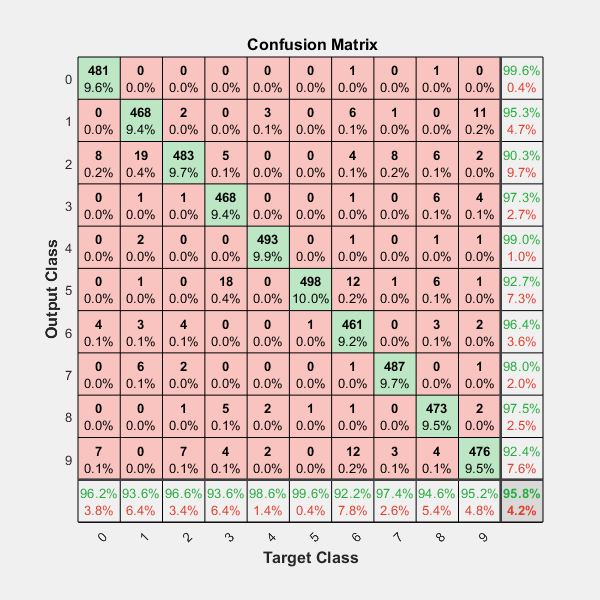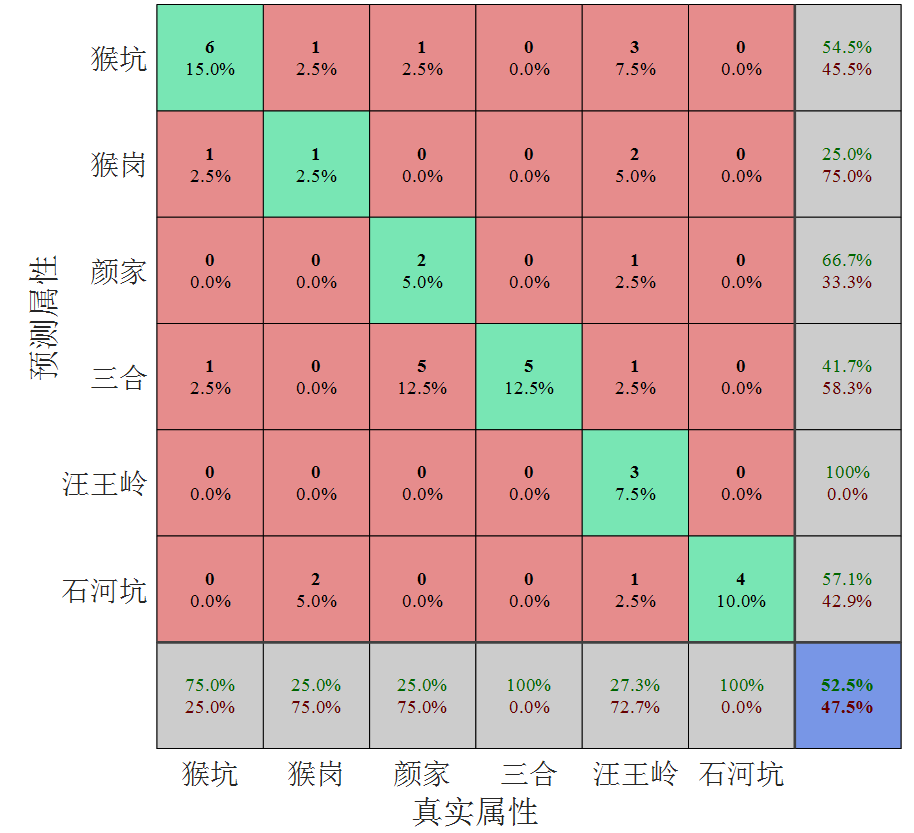• 高维多标签分类matlab knn，svm，随机森林等算法 784维数据 分为10类
• 在使用matlab处理数据标签时， 1，首先要判断这张图片标签是否是符合规则的，对于不符合要舍去。在某些情况下，可能不能完全舍去。那么就需要，根据保留标签的特点，来存储数据。比如 使用case 语句。 2，标签可以...
在使用matlab处理数据标签时，

1，首先要判断这张图片标签是否是符合规则的，对于不符合要舍去。在某些情况下，可能不能完全舍去。那么就需要，根据保留标签的特点，来存储数据。比如 使用case 语句。

2，标签可以过多或者需要融合，可以根据原始图片的特点来融合数据。


展开全文• 内含有大量多标签多类别分类算法和对应的代码，包括MIML_LPT,MIMLBoost,MIMLSVM,MIMLfast,KISAR,MIMLKNN,MLKNN,DMIMLSVM,MIMLMISVM等等，部分代码含有对应的文献，学习多类标分类的好资源。
• 代码解释 maketree函数递归建立树 tree=struct(‘isnode’,1,‘a’,0.0,‘mark’,0.0,...代码可以自动适应不同的分类标签和类别个数 代码 function tree=maketree(D,a) tree=struct('isnode',1,'a',0.0,'mark',...
代码解释
maketree函数递归建立树
tree=struct(‘isnode’,1,‘a’,0.0,‘mark’,0.0,‘child’,{}) 所有分支递归的存在child{}中
函数gan（D）计算D的信息率
代码可以自动适应不同的分类标签和类别个数
代码
function tree=maketree(D,a)
tree=struct('isnode',1,'a',0.0,'mark',0.0,'child',{});%isnode判断是否是分支还是叶子，a表示节点属性，若节点是叶子，a表示分类结果，child是孩子
tree(1).a=1;%给tree分配一个确切的内存
if length(unique(D(:,end)))==1%D中样本属于同一类别
tree.isnode=0;%把tree标记为树叶
tree.a=D(1,end);%把tree的类别标记为D的类别
return
end

if sum(a)==0 ||length(D)==0 %属性划分完毕
tree.isnode=0;%把tree标记为树叶
tree.a=mode(D(:,end));%把tree的类别标记为D出现最多的类别
return
end
for i=1:length(a)
if a(i)==1
if length(unique(D(:,i)))==1
tree.isnode=0;%把tree标记为树叶
tree.a=mode(D(:,end));%把tree的类别标记为D出现最多的类别
return
end
end
end
gain=zeros(length(a),1); %保存每个属性的信息增益
best=zeros(length(a),1); %保存每个属性的最佳划分

for i=1:length(a)
if a(i)==1
t=D(:,i);
t=sort(t);

gain1=zeros(length(t)-1,1);
for j=1:length(t)-1%二分划分
ta=(t(j)+t(j+1))/2;

Df=D(D(:,i)<=ta,:);
Dz=D(D(:,i)>ta,:);
gain1(j)=ent(D)-(ent(Df)*length(Df(:,end))/length(D(:,end))+ent(Dz)*length(Dz(:,end))/length(D(:,end)));
end

[gain(i),j]=max(gain1);
ta=(t(j)+t(j+1))/2;
best(i)=ta;
end
end
[g,m]=max(gain);%选择信息增益最大的属性
D1=D(D(:,m)<=best(m),:);
D2=D(D(:,m)>best(m),:);
a(m)=0;

tree.a=best(m); %建立分支
tree.mark=m;
% disp('****************************')
% tree.a
% tree.mark
tree.isnode=1;
tree.child(1)=maketree(D1,a);
tree.child(2)=maketree(D2,a);

end
function f=ent(D)%计算信息商
l=unique(D(:,end));
if length(D)==0
f=0;
return
end
f=0;
t=zeros(length(l),1);
for i=1:length(D(:,end))
for j=1:length(l)
if D(i,end)==l(j)
t(j)=t(j)+1;
break;
end
end
end
n=length(D(:,end));
for i=1:length(l)
f=f+(t(i)/n)*log2(t(i)/n);
end
f=-f;
end

测试结果
在训练集测试结果精度是0.91
该测试函数是测试随机森林的函数，但不影响测试决策树。
function randomforest()
clc
clear all
T=1;%bagging采样的次数
M = importdata('D:\毕业设计\数据集1\australian.txt');  %读取数据
[sm,sn]=size(M);
% for i=1:sm             %归一化
%     mins=min(M(i,1:sn-1));
%     maxs=max(M(i,1:sn-1));
%     for j=1:sn-1
%         M(i,j)=2*(M(i,j)-mins)/(maxs-mins)-1;
%     end
% end
indices=crossvalind('Kfold',M(1:sm,sn),10); %十折交叉，划分训练集和测试集
testindices=(indices==1); %测试集索引
trainindices=~testindices;%训练集索引
trainset=M(trainindices,:); %获取训练集
testset=M(testindices,:);%获取测试集
[testm,~]=size(testset);
[trainm,trainn]=size(trainset);

predict=zeros(trainm,T);
for t=1:T %开始bagging采样
D=[];%训练集
for i=1:trainm%采样
k=randperm(trainm,1);
D=[D;trainset(k,:)];
end
[~,sn]=size(D);
a=ones(sn-1,1);%属性集合a，1代表该属性未被划分

tree=maketree(D,a);%递归构造简单决策树

for i=1:trainm
treet=tree;
while 1

if treet.isnode==0
predict(i,T)=treet.a;
break;
end
if trainset(i,treet.mark)<=treet.a
treet=treet.child(1);
else
treet=treet.child(2);
end

end
end

end
acc=0;
for i=1:trainm
if trainset(i,end)==mode(predict(i,:))
acc=acc+1;
end
end
acc=acc/trainm

end




展开全文决策树
• 对于多分类问题，如何对预测结果进行可视化是对比分析的关键一步，在实际多分类问题，除了简单展示模型预测精度外，如何分别不同类别之间的预测结果对于分析样本相关性和属性区别具有重要意义，在MATLAB中一般通过...
对于多分类问题，如何对预测结果进行可视化分析是性能对比的关键，在实际多分类问题，除了简单展示模型预测精度外，如何理解不同类别之间的预测结果对于分析样本相关性和属性区别具有重要意义，在MATLAB中一般通过混淆矩阵confusion matrix进行可视化分析，

基本实现函数为：

1. plotconfusion(targets,outputs)

2. plotconfusion(targets,outputs， name)

其中 targets为样本对应实际标签，outputs为所建模型预测样本标签，name为混淆矩阵名称，并非对应属性名称（譬如产地等）,除上述两种方式为，还有一种表达方式是通过单独属性表示预测结果的，没什么应用价值，此处不再介绍。

需要说明的是：targets和outputs两个矩阵不是实际预测模型的1xN矩阵，而是MxN矩阵，其中N表示样本数目，M表示样本属性个数，即类别数或标签数等。常规模型所得1xN预测属性无法直接进行混淆矩阵绘制，需要对单样本属性进行多属性扩展，具体为建立MxN矩阵，该矩阵行表示属性特性，列表示样本，对于单个样本，仅在对应属性对应行向量进行标注（1），该列向量其余位置均为0，这样，原先的矩阵1xN矩阵转换为MxN矩阵。

官方给出的二分类问题为：其中横轴表示真实样本标签、纵轴表示样本预测标签，下、右四块浅灰色表示TP、TN、FP、FN等有关不平衡分布样本评价指标参数。

官方给出的多分类问题为：可以看到，每一个橙色方块内部表示预测错误样本数，主对角线表示预测正确样本数，下、右浅灰色矩形框表示对应样本属性预测准确率。

最后再贴一张自己对太平猴魁茶产地鉴别结果的可视化：我对图中的字体和框图颜色进行了调整。

完结，加油，我的博士梦！

展开全文混淆矩阵 可视化
• MATLAB中的分类器 ...现将其主要函数使用方法总结如下，更细节需参考MATLAB 帮助文件。 设 　训练样本：train_data % 矩阵，每行一个样本，每列一个特征 　训练样本标签：train_label % 列向量

MATLAB中的分类器

MATLAB中分类器有：K近邻分类器，随机森林分类器，朴素贝叶斯，集成学习方法，鉴别分析分类器，支持向量机。现将其主要函数使用方法总结如下，更多细节需参考MATLAB 帮助文件。设　　训练样本：train_data             % 矩阵，每行一个样本，每列一个特征　　训练样本标签：train_label       % 列向量　　测试样本：test_data　　测试样本标签：test_label K近邻分类器 （KNN）mdl = ClassificationKNN.fit(train_data,train_label,'NumNeighbors',1);predict_label   =       predict(mdl, test_data);accuracy         =       length(find(predict_label == test_label))/length(test_label)*100                随机森林分类器（Random Forest）B = TreeBagger(nTree,train_data,train_label);predict_label = predict(B,test_data);  朴素贝叶斯 （Na?ve Bayes）nb = NaiveBayes.fit(train_data, train_label);predict_label   =       predict(nb, test_data);accuracy         =       length(find(predict_label == test_label))/length(test_label)*100;  集成学习方法（Ensembles for Boosting, Bagging, or Random Subspace）ens = fitensemble(train_data,train_label,'AdaBoostM1' ,100,'tree','type','classification');predict_label   =       predict(ens, test_data);  鉴别分析分类器（discriminant analysis classifier）obj = ClassificationDiscriminant.fit(train_data, train_label);predict_label   =       predict(obj, test_data);  支持向量机（Support Vector Machine, SVM）SVMStruct = svmtrain(train_data, train_label);predict_label  = svmclassify(SVMStruct, test_data)


展开全文• MATLAB分类器有：K近邻分类器，随机森林分类器，朴素贝叶斯，集成学习方法，鉴别分析分类器，支持向量机。 现将其主要函数使用方法总结如下，更细节需参考MATLAB 帮助文件。设： 　训练样本 ：train_data 　...
• 现将其主要函数使用方法总结如下，更细节需参考MATLAB帮助文件。 设 　训练样本：train_data%矩阵，每行一个样本，每列一个特征 　训练样本标签：train_label %列向量 　测试样本：test_data 　测试样本标签：test...
• 2.0 得知分类标签常用+1，-1，但是是任意选择的，对于多分类问题，建议选择1，2，3... 3.0 交叉验证寻找最优的c和gamma效果不错，但是用时过长。 4.0 libsvm的详细用法和参数解析：上一页。 1.采用交叉验证法获得的...
• 现将其主要函数使用方法总结如下，更细节需参考MATLAB帮助文件。 设 　训练样本：train_data%矩阵，每行一个样本，每列一个特征 　训练样本标签：train_label %列向量 　测试样本：test_data 　测试样本标签：test...
• 问题：带有标签1,2,3的3级分类.工具：用于MATLAB的LibSVMsvmModel = svmtrain(, , '-b 1 -c -g ');[predLabels, classAccuracy, **probEstimates**] = svmpredict(, , '-b 1');在这一步之后,我得到了前十行的...
• %% 生成txt格式的图像分类标签 path='D:\人工智能\图像识别数据\'; folders=dir(path);% dir是direcory的缩写，是目录的意思，返回的folders是结构体，包含所有的目录和两个特殊的目录，一个是“.”，代表该文件夹...
• 在神经网络和机器学习的结果分析中，常常会用混淆矩阵和ROC曲线来分析识别/分类结果的好坏，而且论文中也经常出现这种图。对于卷积神经网络来说画混淆矩阵很简单，要用到函数plotconfusion，格式为plotconfusion...神经网络 机器学习
• 目前了解到的MATLAB分类器有：K近邻分类器，随机森林分类器，朴素贝叶斯，集成学习方法，鉴别分析分类器，支持向量机。现将其主要函数使用方法总结如下，更细节需参考MATLAB 帮助文件。 设 　训练样本：train...
• 这一篇比较简单，讲的是利用matlab提供的一个工具来生成带标签区域的图片，作为对象检测器模型的训练数据。其实机器学习领域，数据的重要性是大于算法的，但是没有多少人会安安静静成下心来研究怎么收集和处理数据，...
• 目前了解到的MATLAB分类器有：K近邻分类器，随机森林分类器，朴素贝叶斯，集成学习方法，鉴别分析分类器，支持向量机。现将其主要函数使用方法总结如下，更细节需参考MATLAB 帮助文件。 设 　训练样本：train...
• 目前了解到的MATLAB分类器有：K近邻，随机森林，朴素贝叶斯，集成学习方法，鉴别分析，支持向量机。现将其主要函数使用方法总结如下，更细节需参考MATLAB 帮助文件。 设： 训练样本：train_data % 矩阵，每行一...
• 多分类问题中，有一种很实用的分类问题结果统计图。 比如说类别文类问题，那么...我最近也在做多分类问题，要画这样的图，但是发现确实很少有代码，自己画的确实不好看，还牵扯到值的显示和x轴标签的旋转问题，混淆矩阵
• %datas为读入的数据集 labels为读入的标签 %规范化数据 [datas_normal] = premnmx(datas) ; %交叉验证 k =10;%预将数据分成十份 sum_accuracy_svm = 0; [m,n] = size(datas_normal); %交叉验证,使用十折交叉验证 ...
• function [ result ] = TDW_multiclass( ...% 该函数是基于one-vs-one方法的得到的处理类问题的TDW分类器 % trainX 训练样本的特征向量构成的n行m列矩阵，每一行是一个样本 % trainY 训练样本的标签构成的n行...TDW 机器学习
• 随机森林算法的相关资料，参考：https://www.cnblogs.com/maybe2030/p/4585705.html（1）首先制作训练样本，并对样本打分类标签；（2）训练样本，并在训练集上的利用“包外错误率oob”评估模型效果；（注意：训练...
• 多分类问题中，有一种很实用的分类问题结果统计图。...我最近也在做多分类问题，要画这样的图，但是发现确实很少有代码，自己画的确实不好看，还牵扯到值的显示和x轴标签的旋转问题，所以一直自己也没...
• 分类专栏： 传感器融合 智能交通 文章标签MATLAB跟踪器 目标跟踪 传感器融合 trackerGNN 全局最近邻 版权 传感器融合--MATLAB跟踪器介绍 MATLAB通过目标跟踪器可以融合传感器检测到的目标信息，常...# matlab多标签分类matlab 订阅